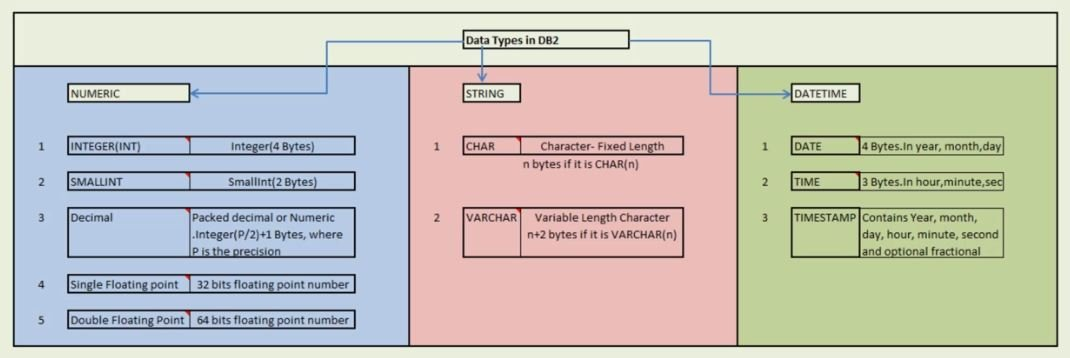# Data types in DB2

## DB2 Data Types

There are three types of Data types:

1. Numeric Data Types
2. String Data Types
3. Datetime Data Types### Numeric Data types

In Numeric Data types we have 5 types of Data Types

i) Integer Data types – Integer occupies 4 bytes in memory and it has 31 precession and the range for this integer is -21474836482+2147493647. It occupies 4 bytes. For the bigger number, we can define INTEGER.

ii) SMALLINT Data types – It is a binary data type integer and the range is -32682+32767. It occupies 2 bytes. For a smaller number, we can define as the SMALLINT.

iii) DECIMAL Data types – Decimal is also called as numeric and can also say this as a packed decimal.

For Example, we have decimal of 10, 2 that means it has total digit as 10 and after the decimal point it has two digits. The range of this decimal is 1-10(power 31) to 10(power31-1). In this packed decimal or numeric, the range is integer p/2+1 byte, where p is precision. It has an implicit decimal point and the position of the decimal point is always determined by the precision and scale of the number.

iv) Single Floating Point Data types – It is also called REAL and the range is -7.2E+75 to 7.2E to 75. It is also used very less it has 32 bits floating number.

v) Double Floating Point Data types – Floating number is 64 bits and the range is -7.2E + 75 to + 7.2E + 75.

### String Data types

In String Data types, we have CHAR and VARCHAR.CHAR is a fixed length. For Example, if I give char(4) than it will occupy 4 byte, n bytes if it is CHAR(n).VARCHAR is variable length character, a number of bytes are n+2 if varchar is defined as VARCHAR(n).

### Datetime Data types

In DateTime we have

i) Date – Date is 4 byte and it has three parts DAY, MONTH, YEAR. (YYYY)A year can occupy a value of 000129999 while MM(momnth0 can occupy a value from 1 to 12 and DD(Day) can occupy 1 to 28 or 1 to 30 or 1 to 31 or 1 to 29, it all depends on the month and year. In memory, it occupies 4 bytes.

ii)Time – In time we have three bytes the first byte is (HH)hour, (SEC)second byte is (MM)minute and the third byte is second.

iii)TimeStamp – It contains year, month, date, hour, minute, second and sometimes it also contains optional fractional second and a few times it also contains a time zone as well.

There are other data types as well, For Example, ex-MIL or null. We cover this in details. We also have row id value which is unique for each row and we can also retrieve the record based on row id as well. So, in data types whatever data types we have in DB2 we will see that if we create an equivalent data type in COBOL we can do that using DCLGEN.